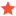what exactly is the rotation system "UR(deg)" and "UR(rad)" in roboDK?cwwstcJunior MemberPosts: 2 Threads: 1 Joined: Oct 2018 Reputation: 0 10-19-2018, 05:06 PM Hello, My question is what exactly is the rotation system "UR(deg)" and "UR(rad)" in roboDK? For example, after I import a UR5 robot from Library, I select UR5 Base frame, selecti reference position with respect to "New Station(1)", and I set all 6 position parameters to Zero.Then I select UR(deg) position system. Now is still all zero. Without adding offset in distance, simplely apply following position parameters: (0,0,0,90,0,0) = Rotation along X-axis for 90 degree, conter-clockwise (right hand rule). (0,0,0,0,90,0) = Rotation along Y-axis for 90 degree, conter-clockwise (right hand rule). (0,0,0,0,0,90) = Rotation along Z-axis for 90 degree, conter-clockwise (right hand rule). So far it is all correct. Then I try the input of (0,0,0,90,90,0), weird rotation appears. According to UR5 rotation rule, it is using intrinsic Z-Y-X order. I would like to see [Assume when was (0,0,0,0,0,0), X-axis points forwards, Y-axis points left and Z-axis points upwards] a result of X-axis points downwards, Y-axis points forwards, and Z-axis points to right. However, what I get is as atttached image. It rotates in a weird manner.I cannot understand how does the rotation work for UR coordinate in RoboDK. If it is not a bug, please explain to me, thank you so much. Attached files: 1) 0,0,0.png : Just approve that the original point of view is correct and normal to Y-Z planes 2) 90,90,0.png : From exactly  same point of view as 0,0,0.png, just by inputting (0,0,0,90,90,0). Find ReplyAlbertModeratorPosts: 1,424 Threads: 1 Joined: Apr 2018 Reputation: 74 10-22-2018, 08:23 PM Hello, Universal Robots uses what they call uvw values to represent the orientation of a pose. These values correspond to the rotation vector and not a Euler angle representation. The norm of this uvw vector is the rotation (seen in radians or degrees, depending on your choice).  This representation is similar to ABB's representation (ABB RAPID programming uses Quaternions). uvw and Quaternions are both an alternative to Euler angles. Euler angles are used in most robot controllers, such as Motoman, Fanuc, KUKA, Staubli, Mecademic, etc. ABB and UR don't use Euler angles.  Any pose in RoboDK has the same menu you see in the following image. This window allows you to see the same transformation in different representations.In any case, no matter the representation you choose for the transformation, the post processor will convert the pose (transformation) to the correct format for your controller. More information here: https://robodk.com/doc/en/Basic-Guide.html#RefFrames https://robodk.com/blog/robot-euler-angles/ (this is not a bug so I'm moving it to general questions) Find ReplycwwstcJunior MemberPosts: 2 Threads: 1 Joined: Oct 2018 Reputation: 0 10-23-2018, 12:22 PM Thank you very much! Your answer is very helpful and useful! Best regards. (10-22-2018, 08:23 PM)Albert Wrote: Hello, Universal Robots uses what they call uvw values to represent the orientation of a pose. These values correspond to the rotation vector and not a Euler angle representation. The norm of this uvw vector is the rotation (seen in radians or degrees, depending on your choice).  This representation is similar to ABB's representation (ABB RAPID programming uses Quaternions). uvw and Quaternions are both an alternative to Euler angles. Euler angles are used in most robot controllers, such as Motoman, Fanuc, KUKA, Staubli, Mecademic, etc. ABB and UR don't use Euler angles.  Any pose in RoboDK has the same menu you see in the following image. This window allows you to see the same transformation in different representations. In any case, no matter the representation you choose for the transformation, the post processor will convert the pose (transformation) to the correct format for your controller. More information here: https://robodk.com/doc/en/Basic-Guide.html#RefFrames https://robodk.com/blog/robot-euler-angles/ (this is not a bug so I'm moving it to general questions) Find Reply